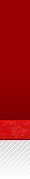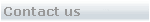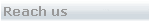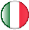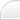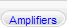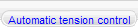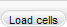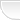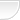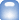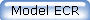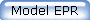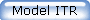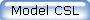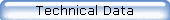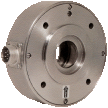Flange mounted load cells "EPR" series are partucularly recommedended for:

-Mounting with sensor rolls having throungh shaft ends

-Mounting both inside and outside machine frame

-Installation in narrow spaces.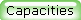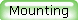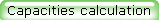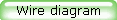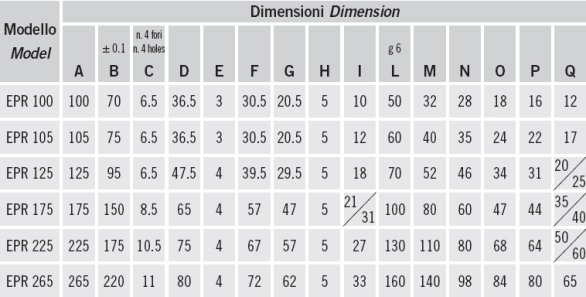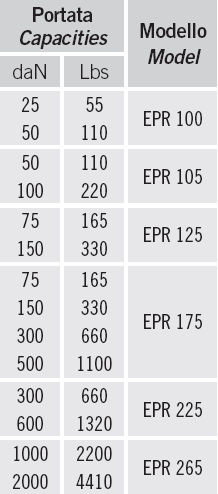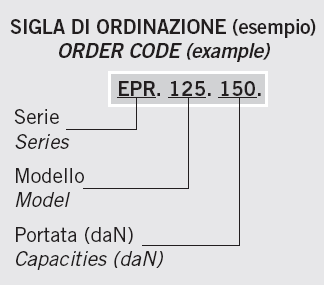MONTAGGIO CELLE IN ESTERNO SPALLE MACCHINA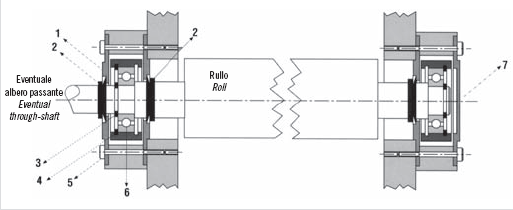MONTAGGIO CELLE IN INTERNO SPALLE MACCHINA1 - Coperchio passante 2 - Tenuta V-RING 3 - Anello SEEGER 4 - Anello SEEGER per foro 5 - Vite di fi ssaggio 6 - CuscinettoB = Angolo tra entrata e uscita laminato Angle between entering and exiting web C = Angolo tra forza risultante Ft ed asse verticale / Angle between tension force Ft and vertical axis Ft = Forza risultante (daN) Force due to tension (daN) T = Tiro massimo (daN) Maximum tension (daN) A = Angolo di avvolgimento rullo Wrape angle →= Riferimento direzione risultante Force direction reference P = Peso rullo (daN) Roll weight (daN) Fc = Max forma di lavoro sulla cella (daN) Maximum force on cell (daN) IMPORTANT: K is a transient overload factor. K is normally between 1.5 and 2. REMARK: We reccomends to apply the load cell as per angle shown in scheme 1 or 2. If possible avoid the use of scheme 3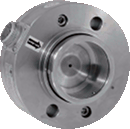Flange mounted load cells "ITR" series maintain the typical characteristics of the "ECR" series with the possibility to have higher load capacities and bigger ball bearing seats.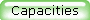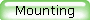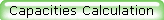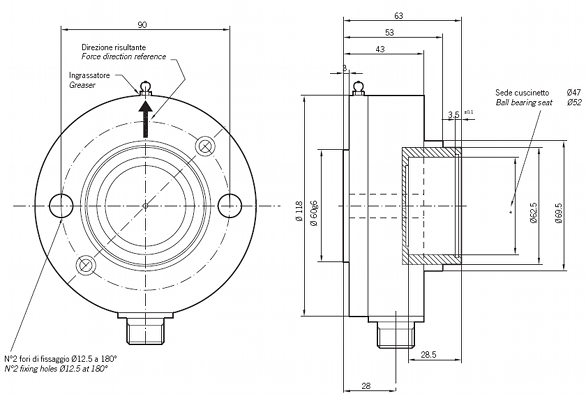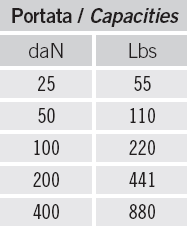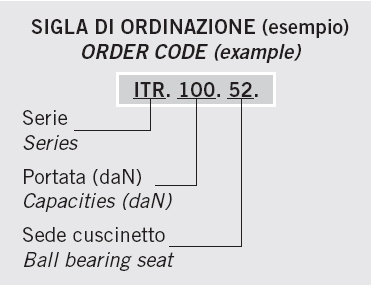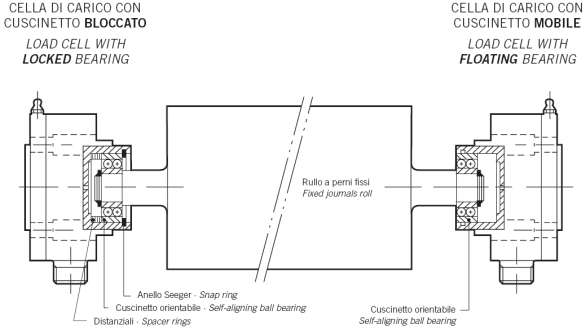B = Angolo tra entrata e uscita laminato Angle between entering and exiting web C = Angolo tra forza risultante Ft ed asse verticale / Angle between tension force Ft and vertical axis Ft = Forza risultante (daN) Force due to tension (daN) T = Tiro massimo (daN) Maximum tension (daN) A = Angolo di avvolgimento rullo Wrape angle →= Riferimento direzione risultante Force direction reference P = Peso rullo (daN) Roll weight (daN) Fc = Max forma di lavoro sulla cella (daN) Maximum force on cell (daN) IMPORTANT: K is a transient overload factor. K is normally between 1.5 and 2. REMARK: We reccomends to apply the load cell as per angle shown in scheme 1 or 2. If possible avoid the use of scheme 3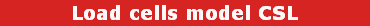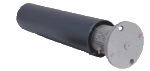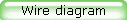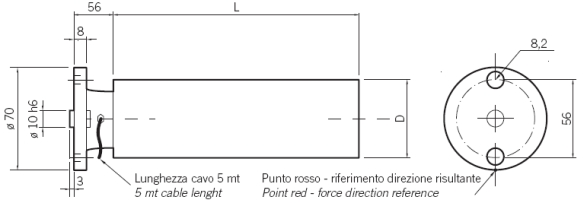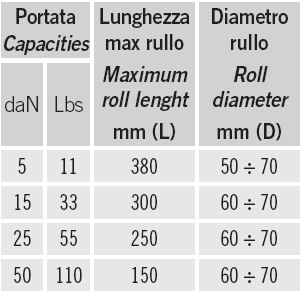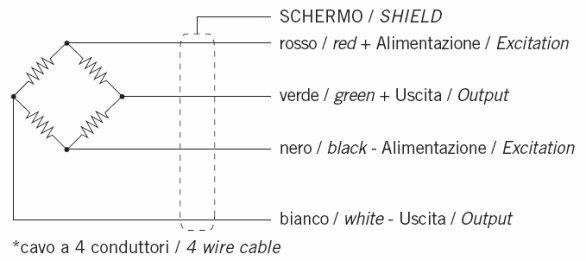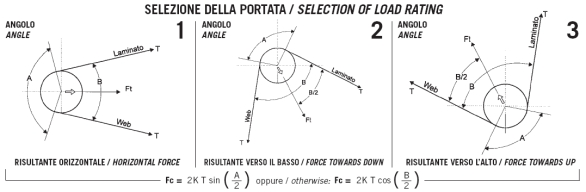IMPORTANT: K is a transient overload factor. K is normally between 1.5 and 2. REMARK: We reccomends to apply the load cell as per angle shown in scheme 1 or 2. If possible avoid the use of scheme 3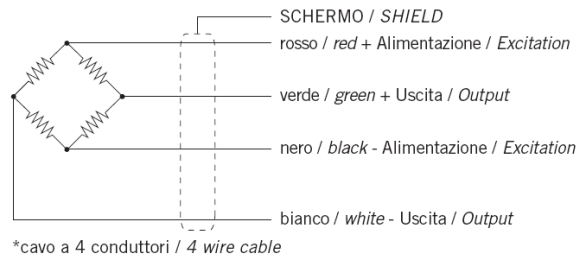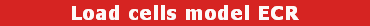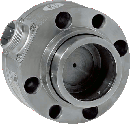Flange mounted load cells "ECR" series are suitable for assembling on a roll sensor end part (extremity).

The standard application provides the fixing on a machine frame or base fixing by means of a "L" profile.

The constructive accuracy and the high metrological performance are able to ensure an extremely precise output signal.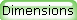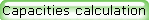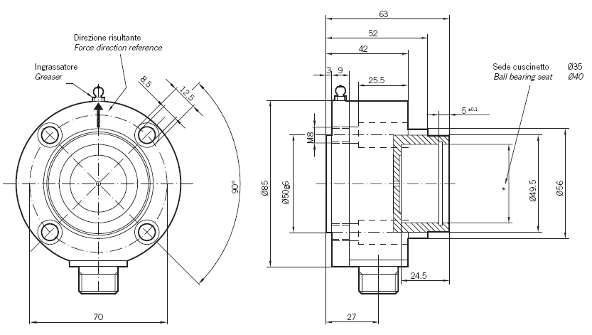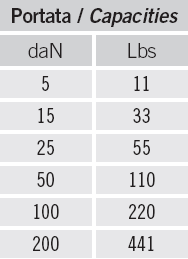B = Angolo tra entrata e uscita laminato Angle between entering and exiting web C = Angolo tra forza risultante Ft ed asse verticale / Angle between tension force Ft and vertical axis Ft = Forza risultante (daN) Force due to tension (daN) T = Tiro massimo (daN) Maximum tension (daN) A = Angolo di avvolgimento rullo Wrape angle →= Riferimento direzione risultante Force direction reference P = Peso rullo (daN) Roll weight (daN) Fc = Max forma di lavoro sulla cella (daN) Maximum force on cell (daN) IMPORTANT: K is a transient overload factor. K is normally between 1.5 and 2. REMARK: We reccomends to apply the load cell as per angle shown in scheme 1 or 2. If possible avoid the use of scheme 3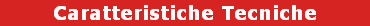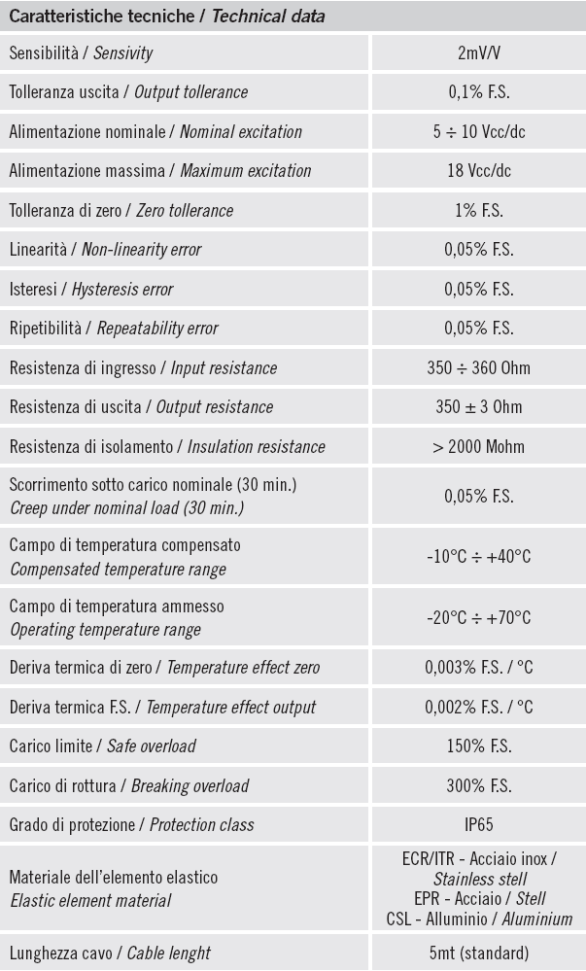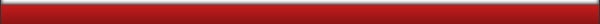RED-Renato Desirò Via Sant'Anna,51  20015 Parabiago (MI) Italy  Tel +39 0331 491452 Fax +39 0331 493591  e-mail: info@red-products.it